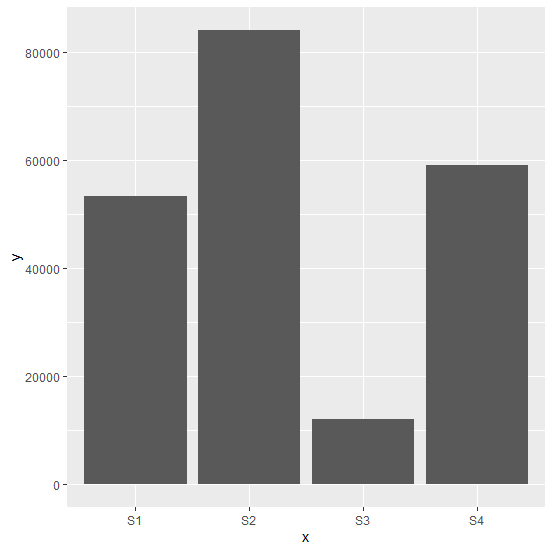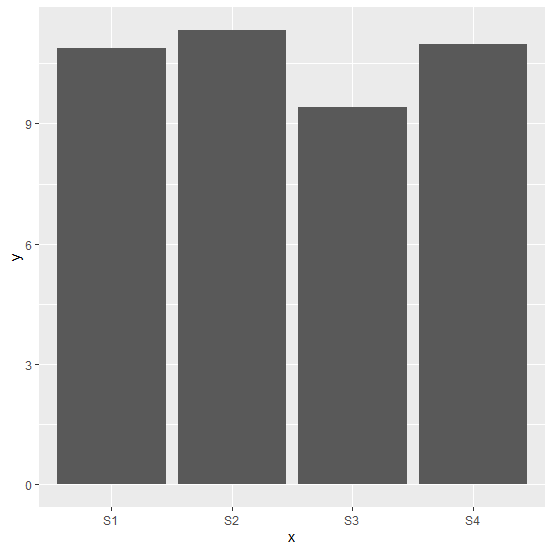# How to create bar plot with log values using ggplot2 in R?

To create the bar plot using ggplot2, we simply need to use geom_bar function and if we want to have the log scale of y variable then it can be set with aes under geom_bar. For example, if we have a data frame called df that contains a categorical column x and a numerical column y then the bar plot with log of y can be created by using the below command −

ggplot(df,aes(x,y))+geom_bar(stat="identity",aes(y=log(y)))

## Example

Consider the below data frame −

Live Demo

> x<-c("S1","S2","S3","S4")
> y<-sample(10000:99999,4)
> df<-data.frame(x,y)
> df

## Output

   x     y
1 S1 53347
2 S2 84208
3 S3 12140
4 S4 59105

Loading ggplot2 package and creating bar chart for data in df −

> library(ggplot2)
> ggplot(df,aes(x,y))+geom_bar(stat="identity")

## OutputCreating bar chart for data in df with log of y −

> ggplot(df,aes(x,y))+geom_bar(stat="identity",aes(y=log(y)))

## Output